#### Introduction to Symmetry, Chemistry tutorial

Introduction

The idea of symmetry is a familiar one: we speak of a shape as "being symmetrical",  "unsymmetrical" or even more symmetrical than some other shapes". Scientifically, we need to specify ideas to symmetry in a more quantitative way. Which of these shapes will we say is more symmetrical?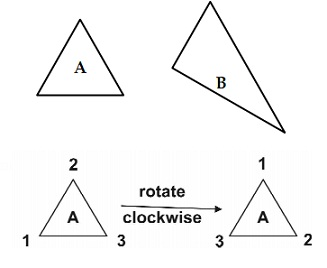Fig: Two structures above are indistinguishable

If you rotate a piece of cardboard shaped like "A" by one third of a turn, the result looks the same as the starting point.

Since the two structures above are indistinguishable, we say that the rotation is a symmetry operation of the shape.

Definition of Symmetry

An object  is  said  to  be  symmetrical  if  on  operated  upon  either  by rotation or reflection on a plane, or inversion through the centre remains indistinguishable. This operation consists of treating the plane as a mirror, which gives an image behind the mirror that is identical to the scene in front of the mirror.

This reflection in the mirror produces a result that is indistinguishable from that existing originally (Atkins, 1982; Babarinde, 2008 & Barrow, 1982). The reflection process is an example of a symmetry operation. It is the symmetry operation associated with a plane of symmetry. The plane of symmetry is an example of an element of symmetry. In formal terms, we say that an object is symmetric through respect to a given mathematical process, if when applied to the object, this operation does not change the object or its appearance. Two objects are symmetric to each other with respect to a given group of operations if one is obtained from the other through several of the operations (and vice versa). Symmetries might as well be originated in living organisms including humans and other animals.

Symmetry Elements and Operations

Each symmetry element will generate symmetry operation. The operations that will be generated are as tabulated below.

Identify (E): Does nothing, it has no effect. All molecules/objects possess the identity operation, that is, posses E.  E has the same importance as the number 1 does in multiplication (E coming from the German word "Einheit", meaning unity). E is needed in order to define inverses.

Proper axis of rotation (Cn): Rotation through angle θ =360o/n C2 = 180° rotation, C3 = 120° rotation, C4 = 90° rotation, C5 = 72° rotation,

C6 = 60° rotation, etc

Rotation of H2O about the axis shown by 180° (C2) provides the similar molecule back.

Therefore, H2O possess the C2 symmetry element.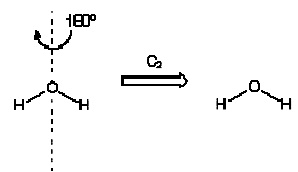Fig: symmetry element

Though, rotation by 90° about the similar axis doesn't provide back the identical molecule Therefore H2O does NOT possess a C4 symmetry axis.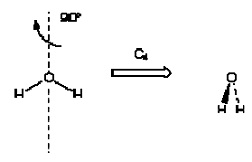Fig: rotation axis of symmetry

BF3 posses aC3 rotation axis of symmetry. In each case both directions of rotation must be considered.Fig: square planar

XeF4 is square planar. It has four dissimilar C2 axes. It also has a C4 axis coming out of the page termed the principle axis since it has the major n.

By convention, the principle axis is in the z-direction. When there is more than one axis in a molecule, one of them is the principal axis that has the largest value of n. The principal axis is perpendicular to the plane of the molecule. When there is only one axis of rotation that axis constitutes the principal axis. For an equilateral triangle there are 2C3 and 3C2 axes. Since 3 are greater than 2 then the principal axis is C3.

Mirror plane (  ):  Reflection through plane. If reflection about a mirror plane provides the similar molecule/object back, than there is a plane of symmetry ( ). If plane contains the principle rotation axis (i.e., parallel), it is a vertical plane (v). If plane is perpendicular to the principle rotation axis, it is a horizontal plane (h). If plane is parallel to the principle rotation axis, but bisects angle between 2C2 axes, it is a diagonal plane (d). H2O posses 2v mirror planes of symmetry since they are together parallel to the principle rotation axis (C2).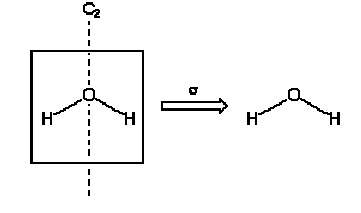Fig: two planes of symmetry

XeF4 has 2 planes of symmetry parallel to the principle rotation axis: v XeF4 has 2 planes of symmetry parallel to the principle rotation axis and bisecting the angle between 2C2 axes: d. XeF4 has one plane of symmetry perpendicular to the principle rotation axis: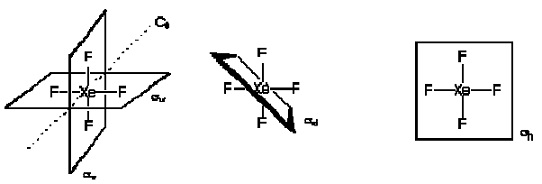Fig; perpendicular to the principal axis

The mirror  plane  that  is  perpendicular  to  the  principal  axis  is  the horizontal plane (h ). The mirror plane that is parallel to the principal axis is the vertical plane (v). The plane that bisects the conflicting angles of a square and as well parallel to the principal axis in the dihedral plane (d).

Inversion (i): Inversion through the centre.

The component that corresponds to this operation is a centre of symmetry or inversion centre. The operation is to move each atom in the molecule in a straight line through the inversion centre to the opposite side of the molecule.Fig: Inversion

Improper axis of rotation (Sn): Rotation through axis Cn followed via reflection in a plane. There is n-fold rotation followed through reflection during mirror plane perpendicular to rotation axis. A body such as equilateral triangle has improper axis of rotation Sn. It can be rotated through C3 at the centre of the triangle and there is as well a plane on the paper. The principal axis C3 is perpendicular to the plane of the paper. A blend of the proper axis of rotation and the mirror plane provides the improper axis of rotation Sn.

S3                hC3

Conventionally, the right hand side of the above equation is read as proper rotation C3 followed via mirror plane h. When there are 2 inversions then the consequence will be the identity (E). 2 E

For invention: in = i when n is odd.

in = E when n is even.

Reflection twice produces the identity.

n when n is odd. ,  E   when n is even.

Tutorsglobe: A way to secure high grade in your curriculum (Online Tutoring)